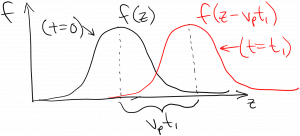Laser Photonics Properties of the scalar wave equation solutions

# Properties of the scalar wave equation solutions

How does the solution$E_x = f(z - v_p t)$

behave?  Well, we know that subtracting a number from the argument of a function shifts that function to the right.  For example, suppose that$g(x)$ is a Gaussian function of$x$.  Replacing$x$ with$(x - d)$ will shift the function to the right by a distance$d$:So when we’re considering$f(z - v_p t)$, if we were to plot this function vs.$z$ at$t = 0$ and also at$t = t_1$,  the$t = t_1$ version will be the same as the$t = 0$ version except shifted to the right by a distance$v_p t_1$.  If$f(x, t = 0)$ is a Gaussian, then, the plot of$f(z, t)$ at the two times would look like:Since the function has moved in the positive$z$ direction by a distance$v_p t_1$ in time$t_1$, it must be moving at a speed of$v_p$.  In fact, we call the quantity$v_p$ the phase velocity.  The solutions to the wave equation are waves, and$v_p$ is the speed at which the wave is moving.

I mentioned briefly that there is another possible solution to the scalar wave equation, namely:$E_x = f(z + v_p t)$

Hopefully you can see that this will be a wave moving to the left (negative$z$ direction) with speed$v_p$.  In fact, if we went back to the more general form of the Helmholtz equation, we would find solutions moving in all possible directions in 3d space at speed$v_p$.